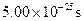Chapter 33, Problem 7PE

Chapter
Section
Textbook Problem

Suppose a W− created in a bubble chamber lives forWhat distance does it move in this time if it is traveling at 0.900 c? Since this distance is too short to make a track, the presence of the W− must be inferred from its decay products. Note that the time is longer than the given W− lifetime, which can be due to the statistical nature of decay or time dilation.

To determine

The distance travelled by W particle created in a bubble chamber.

Explanation

Given info:

W particle created in a bubble chamber lives for 5×1025 s and it is travelling at a speed of 0.900c.

Formula used:

If v is the speed and t be the time taken by a particle, then distance travelled by the particle, =vt

Calculation:

t=5×1025 s and v=0.900c=0.900×3×108=2

Still sussing out bartleby?

Check out a sample textbook solution.

See a sample solution

The Solution to Your Study Problems

Bartleby provides explanations to thousands of textbook problems written by our experts, many with advanced degrees!

Get Started

Describe how Dalton obtained relative atomic weights.

General Chemistry - Standalone book (MindTap Course List)

Little fat digestion takes place in the stomach. T F

Nutrition: Concepts and Controversies - Standalone book (MindTap Course List)

What is meant by the term semiconservative replication?

Chemistry for Today: General, Organic, and Biochemistry